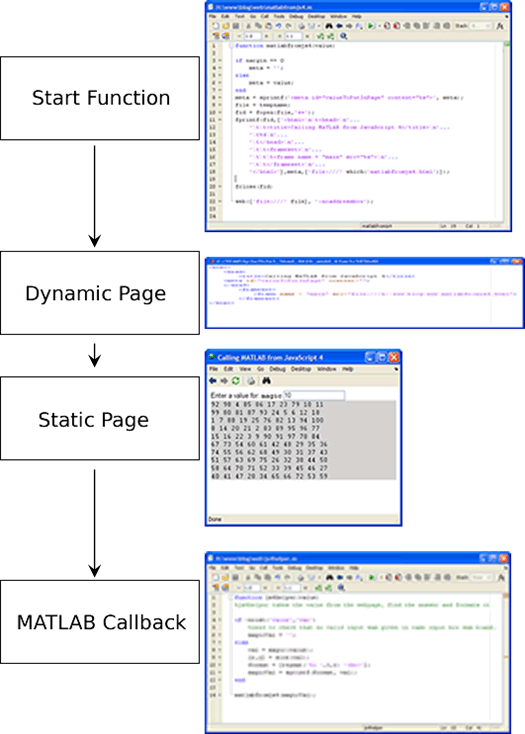Interactive web pages in MATLAB, part 4

A few weeks ago Ashwin commented on my Interactive web pages in MATLAB series from last year asking about computing data in MATLAB and putting back into a web page. I didn’t take the time back then how to explain it because it’s not the most straightforward and requires a bit of javascript.

So without further ado, I’m going to demonstrate one way to take data round-trip from a web page through MATLAB (all running in the same MATLAB session). The main piece of this method is that I am going to have a start function which dynamically creates a web page which loads the desired workspace data. This dynamic page then loads a static page, which is the GUI the user interacts with. This page calls a callback function which evaluates the data from the page and starts the cycle over again. Here is the data flow.The main function
The purpose of the main function is to be the entry point to the web GUI. It takes data passed in and puts it into a dynamically created web page, that loads our static page. This process could be simplified by creating the whole html part dynamically, but that looses the benefit of using a better html-editing tool to create a nice looking web page (this is not an example of that).

The tricky part is that we are storing our value to be used in the main GUI in a meta tag. This allows us to look it up from a javascript method in the main page. The main page is loaded in a frame so that it still has access to the meta tag created here. Otherwise we would have to write that meta tag directly to the main page.

function matlabfromjs4(value)
%matlabfromjs4 load a web gui to print out the result of the magic command

if nargin == 0
%if no arguments (i.e. the first time) then do not load any data
meta = '';
else
%otherwise put it in a meta tag
meta = value;
end
meta = sprintf('<meta id="valueToPutInPage" content="%s">', meta);

%dynamically generate the html and write it out a temporary file
file = tempname;
fid = fopen(file,'w+');
'\t\t<title>Calling MATLAB from JavaScript 4</title>\n'...
'\t%s\n'...
'\t\t<frameset>\n'...
'\t\t\t<frame name = "main" src="%s">\n'...
'\t\t</frameset>\n'...
'</html>'],meta,['file:///' which('matlabfromjs4.html')]);
%matlabjs4.html is the main web gui
fclose(fid);

The GUI page
When loaded in the web browser, this page sets the meta content and loads the matlabfromjs4.html into the browser. This page onload queries the value of that tag and writes it into a special div. This page also has a form element that when a value is typed in the box, it will ask MATLAB to do a computation based on that value (in this case magic) and what will refresh the page.

<html>
<script lang="text/javascript">
function calculatemagic() {
var magicValue = document.getElementById("magic").value;
document.location="matlab:js4helper("+ magicValue +");";
}
var pval = parent.document.getElementById("valueToPutInPage");
return pval.content;
}
</script>
<body>
Enter a value for:
<tt><b>magic</b></tt> <input id="magic" onblur="calculatemagic()">
</body>
</html>

The calculateMagic javascript function uses the previously described matlab: operator to call our helper function js4helper.
js4helper
The helper function calculates the output of the magic function and formats the output is a displayable in html string, and calls our main function again with the string, regenerating the frame page, starting the whole process over.

function js4helper(value)
%js4helper takes the value from the webpage, find the answer and formats it

if ~exist('value','var')
%need to check that no valid input was given in case input box was blank.
magicVal = '';
else
val = magic(value);
[r,c] = size(val);
format = [repmat('%i ',1,r) '<br>'];
magicVal = sprintf(format, val);
end
matlabfromjs4(magicVal);

That’s the basics of creating dynamic html GUIs in MATLAB. For extra credit I’m leaving you with two assignments:
1. Use base64 encoding to add an image to the web page without having to create an image file.
2. Use ActiveX on Windows to hook a web page running in IE up to a MATLAB session.